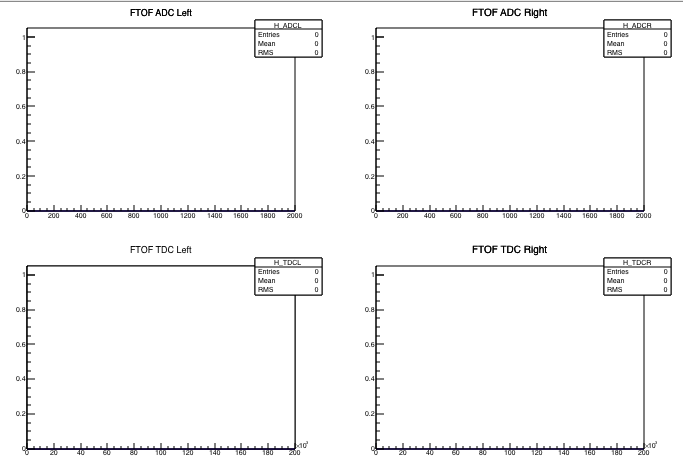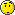Can someone help me to modified this code to have 4 histograms in one sheet
i did , and i got this

So, I have TO have four Histograms in each canvas

Tdc0 and Tdc 1 Left& right
.
.
.
.
Tdc8 and Tdc9 Left&right

{
TFile *f = TFile::Open(“MyData.root”, “RECREATE”);
TTree *t = new TTree(“t”, “My Data tree”);
TH1F *h_tdc = new TH1F(“h_tdc”, “TDC histogram;tdc value;counts”,
4096, 0, 4096);
4096, 0, 4096);
#if 1 /
0 or 1 /
t->Project(“h_tdc”, “tdc”);
#else /
0 or 1 /
t->Draw(“tdc >> h_tdc”, “”, “goff”);
#endif /
0 or 1 */
f->Write();

TCanvas *c = new TCanvas(“c”, “c”);
c->Divide(2, 2);
c->cd(1);
h_tdc->Draw();
c->cd(2);
c->cd(0);pls any kind of help[code]{
TFile *f = TFile::Open(“MyData.root”, “RECREATE”);
TTree *t = new TTree(“t”, “My Data tree”);

for (Int_t i = 0; i < 10; i++) {
h_tdc[i] = new TH1F(TString::Format(“h_tdc_%d”, i),
TString::Format(“TDC %d;tdc value;counts”, i),
4096, 0, 4096);
4096, 0, 4096);
}

for (Int_t i = 0; i < 10; i++) {
#if 1 /* 0 or 1 /
t->Project(TString::Format(“h_tdc_%d”, i),
TString::Format(“tdc[%d]”, i));
#else /
0 or 1 /
t->Draw(TString::Format(“tdc[%d] >> h_tdc_%d”, i, i), “”, “goff”);
#endif /
0 or 1 */
}

f->Write();

TCanvas c = new TCanvas(“c”, “c”, 750, 600);
c->Divide(5, 4);
#if 1 /
0 or 1 /
for (Int_t i = 0; i < 5; i++) {
c->cd(i + 11); gPad->SetLogy(1); h_tdc[(i + 5)]->Draw();
}
#else /
0 or 1 /
for (Int_t i = 0; i < 10; i++) {
}
#endif /
0 or 1 */
c->cd(0);
}[/code]

this code doesn’t work

i want to be c(2, 2) and this one is (5,4)…
( adc 0, adc1 , tdc 0, tdc 1 ) in one Canvas

Didn’t your mum teach you that you’re actually supposed to do your homework yourself?i am new in Root and I tried to learn it by myself, no one teach me, so that why i didn’t know that much in Root. If u don’t want to help me, it is ok but be polite, and thank you gain for your kind help